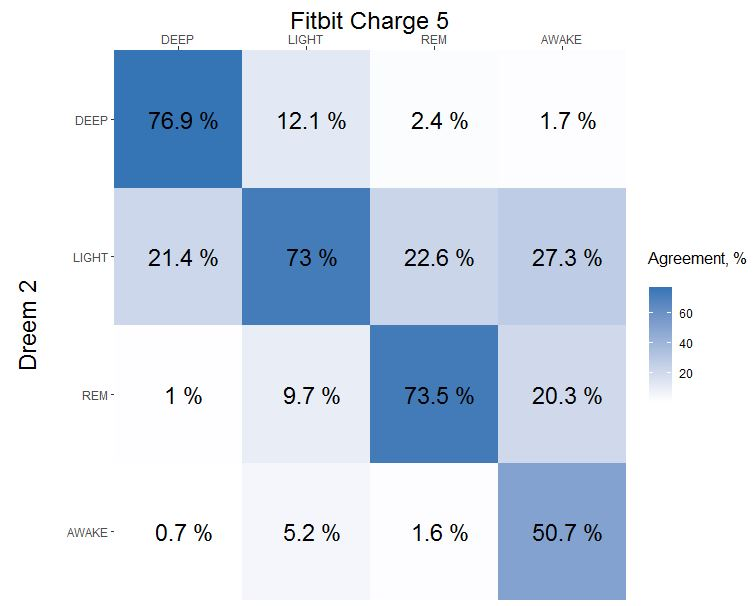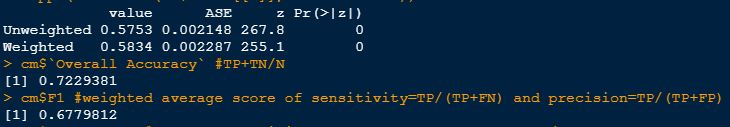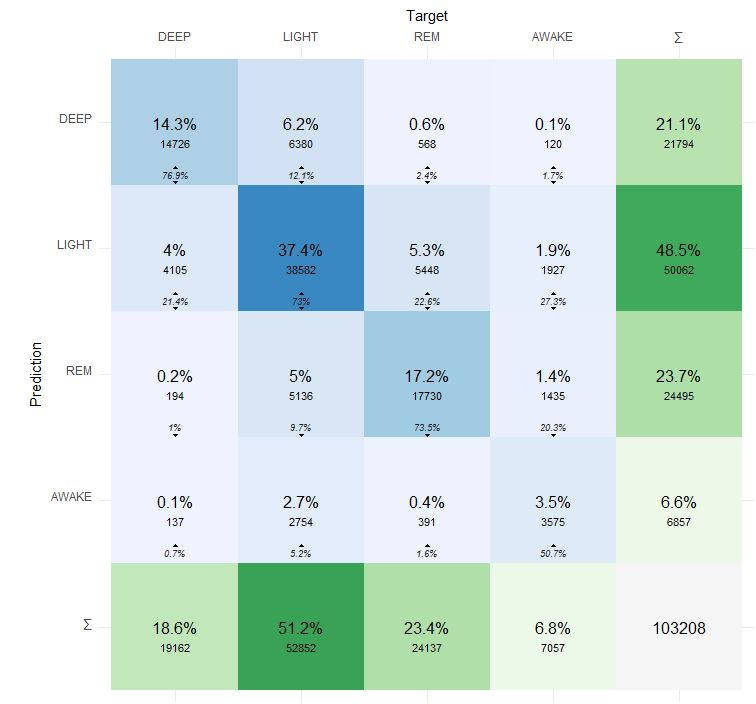Quantified Self Experiments / Accuracy - Fitbit Charge 5 vs Dreem 2 EEG sleep staging

## Abstract

#### What did i do?

I've measured my sleep data by using Fitbit Charge 5 and Dreem 2 EEG.

#### How did i do it?

I've used confusion matrix to reveal agreement between 2 devices.

#### What did i learn?

Fitbit Charge 5 sleep staging looks same as Charge 4 with moderate agreement ~75% to EEG device.
Here is final agreement (diagonal line) if you dont have time to read:Update at 2022-01-23 added 93 more days

## Introduction

Sleep stages data may reveal some sleep disorders and may be used for sleep quality and quantity asessment. Fitbit Charge 5 sleep algorithms determine sleep stages by using non-eeg data (hr, hrv, accelerometer, skin temperature) and accuracy is questionable.

The purpose of this experiment (n=1) is to compared hypnogram data between wristworn device and eeg headband.

## Materials & Methods

#### Participants

Adult male anthropometrics was described in previous article.

#### Experimental design

From 2020-10-06 to 2022-01-22 sleep quality and quantity was assessed by Dreem 2 EEG Headband which was validated against gold standard PSG. At same time Fitbit Charge 5 was used and sleep data was collected. There were 14 nights with data from both devices.

## Results

To compare sleep accuracy i've decided to build confusion matrix. Hypnograms were compared on same resolutions. There were total 112 hours of data and i've decided not to build confidence intervals because of large enough dataset.
Lets build a simplified confusion matrix plot:How to read confusion matrix? Columns belongs to Fitbit Charge 5, rows belongs to Dreem 2, diagonal is agreement between both devices. In a 1st column we can see that 77% of Fitbit DEEP sleep was recognized as DEEP sleep by Dreem 2, 21% of Fitbit DEEP was recognized as LIGHT by Dreem 2 and only 1% of Fitbit DEEP was actually REM. Sum of each column is a 100%.

• 77% of DEEP was detected, misidentified part goes mostly to LIGHT (21%)
• 73% of LIGHT was detected, misidentified part split between REM (10%) and DEEP (12%)
• 73% of REM was detected, misidentified part goes mostly to LIGHT (23%)
• 51% of AWAKE was detected, misidentified part goes mostly to LIGHT (27%).

Cohen's Kappa is 0.58 which is a near moderate agreement:Overall accuracy is 0.72 and F1 is 0.68 which looks ok (both are measures of agreement).

Here is more detailed confusion matrix if anyone interested:Prediction is Dreem 2 and Target is Fitbit Charge 5.

Fitbit Charge 5 does not over/underestimate sleep stages if we look at proportions:

 Fitbit Charge 5 Dreem Difference DEEP 18.6% 21.1% slight LIGHT 51.2% 48.5% slight REM 23.4% 23.7% none AWAKE 6.8% 6.6% none

Percentage base is Time in Bed = DEEP + LIGHT + REM + AWAKE.

Anyway, even average data is impressing and catch sleep composition proportions - this data of less interest because does not require stages predicted at correct time as at previous chart.

## Discussion

This data analysis suggests moderate accuracy of sleep staging from Fitbit Charge 5. But only half of awake time was detected at right time, meaning total sleep time (DEEP + LIGHT + REM), which is of large interest, is inaccurate.

Compared to Oura and Withings devices Fitbit Charge 5 accuracy is impressive, but still far from EEG. Charge 5 performs pretty same compared to Charge 4 and I'm going to switch to Charge 5 over 4.

I'll ignore and will not use Fitbit Charge sleep staging data and TST in my experiments / data analysis for now (even 75% agreement isnt enough and introduce some noise). I maybe use it in a future when i have pretty big dataset which may help to overcome the noise.
In a present time i cant find a non-eeg devices with acceptable sleep staging.

## Data availability & Information

Welcome for questions, suggestions and critics in comments below.

Original unmodified (exported) raw data for fitbit is here and for dreem is here.

```dreem <- read.csv("https://blog.kto.to/uploads/dreem-20-01-2022.csv", skip = 5, sep = ';', header = TRUE)library(jsonlite)library(dplyr)rawfitbit <- bind_rows( fromJSON("https://blog.kto.to/uploads/fc5-v2-sleep-2021-10-05.json"), fromJSON("https://blog.kto.to/uploads/fc5-sleep-2021-11-04.json"), fromJSON("https://blog.kto.to/uploads/fc5-sleep-2021-12-04.json"), fromJSON("https://blog.kto.to/uploads/fc5-sleep-2022-01-03.json"))#process dreem hypnogramdreem <- dreem[!is.na(dreem\$Type),]library(stringr)dreem\$Hypnogram <- str_replace(dreem\$Hypnogram, "\\[", "")dreem\$Hypnogram <- str_replace(dreem\$Hypnogram, "\\]", "")dreem\$Hypnogram <- str_replace_all(dreem\$Hypnogram, "WAKE", "4")dreem\$Hypnogram <- str_replace_all(dreem\$Hypnogram, "REM", "3")dreem\$Hypnogram <- str_replace_all(dreem\$Hypnogram, "Light", "2")dreem\$Hypnogram <- str_replace_all(dreem\$Hypnogram, "Deep", "1")dreem\$HypnogramList <- str_split(dreem\$Hypnogram, ",")dreem <- dreem[dreem\$Start.Time != "2021-08-18T23:01:04+07:00",] #battery went out in the middle of nightdreem <- dreem[dreem\$Start.Time != "2021-10-01T00:15:49+07:00",] #battery went out in the middle of nightdreem <- dreem[dreem\$Start.Time != "2021-10-14T23:15:49+07:00",] #battery went out in the middle of nightdreem <- dreem[dreem\$Start.Time != "2022-01-13T23:27:38+07:00",] #battery went out in the middle of nightdreem <- dreem[dreem\$Start.Time != "2021-12-17T23:00:51+07:00",] #battery went out in the middle of night library(lubridate)dreem\$datetime <- ymd_hms(dreem\$Start.Time)dreems <- dreemlibrary(iterators); library(parallel); library(foreach);library(doParallel); registerDoParallel(cores=12)results <- foreach(i=1:nrow(dreems), .combine='rbind', .multicombine=TRUE, .packages = "lubridate") %dopar% {  ddf = NULL  for (j in 1:length(dreems\$HypnogramList[i][]))  {    stage <- as.numeric(dreems\$HypnogramList[i][][j])    datetime <- dreems\$datetime[i] + 30 * (j - 1)    res <- c(datetime = datetime, stage = stage)    ddf = rbind(ddf, res)  }  return(ddf)}dreem_data <- as.data.frame(results)row.names(dreem_data) <- NULLsummary(dreem_data)#process fitbit hypnogramfitbit <- data.frame(rawfitbit)fitbit\$datetime <- ymd_hms(fitbit\$startTime)fitbits <- fitbit[1,]fitbits <- fitbitfitbit_results <- foreach(i=1:nrow(fitbits), .combine='rbind', .multicombine=TRUE, .packages = "lubridate") %dopar% {  fdf = NULL  data <- as.data.frame(fitbit\$levels\$data[i])  for(j in 1:nrow(data))   {    stage <- data\$level[j]    for(k in 1:round(data\$seconds[j]/30)) #downsample to 30-sec like dreem    {      datetime <- ymd_hms(data\$dateTime[j]) + (k - 1)*30      res <- c(datetime = datetime, stage = stage)      fdf = rbind(fdf, res)     }  }  return(fdf)}fitbit_data <- as.data.frame(fitbit_results)row.names(fitbit_data) <- NULLfitbit_data\$stage <- str_replace_all(fitbit_data\$stage, "wake", "4")fitbit_data\$stage <- str_replace_all(fitbit_data\$stage, "rem", "3")fitbit_data\$stage <- str_replace_all(fitbit_data\$stage, "light", "2")fitbit_data\$stage <- str_replace_all(fitbit_data\$stage, "deep", "1")fitbit_data\$stage <- as.numeric(fitbit_data\$stage)fitbit_data\$datetime <- as.numeric(fitbit_data\$datetime) - 7*3600summary(fitbit_data)#round datas for both hypnogramsperiod <-  "30s"fitbit_data\$dt <- as.POSIXct(fitbit_data\$datetime, origin="1970-01-01 00:00:00")fitbit_data\$period <- round_date(fitbit_data\$dt, period)dreem_data\$dt <- as.POSIXct(dreem_data\$datetime, origin="1970-01-01")dreem_data\$period <- round_date(dreem_data\$dt, period)#merge datamerged_hypnogram <- inner_join(as.data.frame(fitbit_data[,c("period","stage")]), as.data.frame(dreem_data[,c("period","stage")]) , by = c("period" = "period"))colnames(merged_hypnogram) <- c("datetime","fitbit","dreem")merged_hypnogram <- merged_hypnogram[!is.na(merged_hypnogram\$fitbit),]merged_hypnogram <- merged_hypnogram[!is.na(merged_hypnogram\$dreem),]merged_hypnogram\$dreem[merged_hypnogram\$dreem == 1] <- "DEEP";merged_hypnogram\$dreem[merged_hypnogram\$dreem == 2] <- "LIGHT";merged_hypnogram\$dreem[merged_hypnogram\$dreem == 3] <- "REM";merged_hypnogram\$dreem[merged_hypnogram\$dreem == 4] <- "AWAKE";merged_hypnogram\$fitbit[merged_hypnogram\$fitbit == 1] <- "DEEP";merged_hypnogram\$fitbit[merged_hypnogram\$fitbit == 2] <- "LIGHT";merged_hypnogram\$fitbit[merged_hypnogram\$fitbit == 3] <- "REM";merged_hypnogram\$fitbit[merged_hypnogram\$fitbit == 4] <- "AWAKE";category_order <- c("DEEP", "LIGHT", "REM", "AWAKE") merged_hypnogram\$fitbit = factor(merged_hypnogram\$fitbit, levels = category_order)merged_hypnogram\$dreem = factor(merged_hypnogram\$dreem, levels = category_order)library(cvms)cm <- confusion_matrix(targets = as.factor(merged_hypnogram\$fitbit), predictions = as.factor(merged_hypnogram\$dreem))library(vcd)Kappa(as.matrix(cm\$Table[], rownames = F))cm\$`Overall Accuracy` #TP+TN/Ncm\$F1 #weighted average score of sensitivity=TP/(TP+FN) and precision=TP/(TP+FP)cm\$Prevalence #how often positive events occurred (TP + FN) / Np <- plot_confusion_matrix(cm\$`Confusion Matrix`[],                            place_x_axis_above = T,                           add_row_percentages = F,                           add_col_percentages = T,                           rotate_y_text = F,                           class_order = c("AWAKE", "REM", "LIGHT", "DEEP"),                           add_sums = T)pcm <- table(merged_hypnogram\$fitbit,merged_hypnogram\$dreem)cm <- cm / rowSums(cm)cm <- as.data.frame(cm, stringsAsFactors = TRUE)cm\$Var2 <- factor(cm\$Var2, rev(levels(cm\$Var2)))library(ggplot2)ggplot(cm, aes(Var1, Var2, fill = round(100*Freq,1))) +  geom_tile() +  geom_text(aes(label = paste(" ", round(100*Freq,1),"%")),  size=6) +  scale_x_discrete(expand = c(0, 0),position = 'top') +  scale_y_discrete(expand = c(0, 0),position = 'left') +   scale_fill_gradient(low = "white", high = "#3575b5") +  labs(x = "Fitbit Charge 5", y = "Dreem 2", fill = "Agreement, %") +   theme(legend.title = element_text(size = 12, margin = margin(0, 20, 10, 0)),        axis.title.x = element_text(margin = margin(20, 20, 20, 20), size = 18),        axis.title.y = element_text(margin = margin(0, 20, 0, 10), size = 18))```

#### Statistical analysis

RStudio version 1.3.959 and R version 4.0.2. Cohen's Kappa interpretation reference